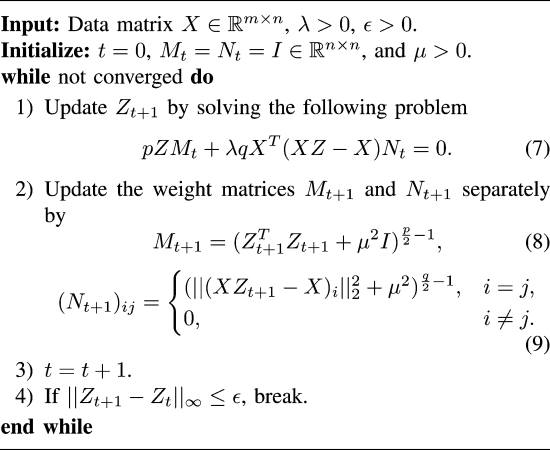# Smoothed Low Rank and Sparse Matrix Recovery by Iteratively Reweighted Least Squared Minimization### Abstract

This paper presents a general framework for solving the low-rank and/or sparse matrix minimization problems, which may involve multiple nonsmooth terms. The iteratively reweighted least squares (IRLSs) method is a fast solver, which smooths the objective function and minimizes it by alternately updating the variables and their weights. However, the traditional IRLS can only solve a sparse only or low rank only minimization problem with squared loss or an affine constraint. This paper generalizes IRLS to solve joint/mixed low-rank and sparse minimization problems, which are essential formulations for many tasks. As a concrete example, we solve the Schatten-p norm and ℓ 2,q -norm regularized low-rank representation problem by IRLS, and theoretically prove that the derived solution is a stationary point (globally optimal if p, q ≥ 1). Our convergence proof of IRLS is more general than previous one that depends on the special properties of the Schatten-p norm and ℓ 2,q -norm. Extensive experiments on both synthetic and real data sets demonstrate that our IRLS is much more efficient.

Type
Publication
IEEE Transactions on Image Processing